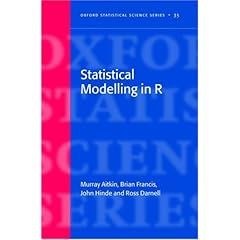# Modeling : R Code,Books and DocumentsFebruary 14, 2009
854 Views

Here is an equivalent of Proc Genmod in R . If the SAS language code is as below- PROC GENMOD DATA=X; CLASS FLH; MODEL BS/OCCUPANCY = distcrop distfor flh distcrop*flh /D=B LINK=LOGIT TYPE3; RUN;   Then the R language equivalent would be : glm(bs/occupancy ~ distcrop*flh+distcrop, […]

Here is an equivalent of Proc Genmod in R .

If the SAS language code is as below-

PROC GENMOD DATA=X;
CLASS FLH;
MODEL BS/OCCUPANCY = distcrop distfor flh distcrop*flh /D=B LINK=LOGIT
TYPE3; RUN;

Then the R language equivalent would be :

glm(bs/occupancy ~ distcrop*flh+distcrop,
family=binomial(logit), weights=occupancy)
where flh needs to be a factor

Credit to Peter Dalgaard from the R-Help List

Peter is also author of the splendid standard R bookSpeaking of books – Here is one R book I am looking /waiting forA similar named free document ( Introduction to statistical modelling in R by P.M.E.Altham, Statistical Laboratory, University of Cambridge)  is available here –

http://www.statslab.cam.ac.uk/~pat/redwsheets.pdf

It is a pretty nice reference document if Modelling is what you do, and R is what you need to explore.It was dated 5 February 2009, so its quite updated and new.You can also check Dr Altham’s home page for a lot of R resources.